ChemTalk

# Wave Functions and Probability Density## Core Concepts

In this article, you will learn about wave functions, an important function that describes the location of an electron in space! This article will also cover how the wave function is calculated, how it relates to probability density, and how it ties into atomic orbitals. Although it is an extremely complex topic, it is an important building block to our understanding of an atom’s electron distribution and energy of electrons today.

## Background of Wave Functions

Previous scientific advancements, such as Young’s double slit experiment, and the photoelectric effect, led scientists to conclude that light is both a wave and a particle. (This phenomenon is also called wave-particle duality.) Louis de Broglie extended this theory to matter, proposing that matter also exhibits wave-particle duality. However, it was Erwin Schrödinger who found a way to express these wave-like properties of electrons using his Schrödinger equation.

## Schrödinger’s Equation

When solved, the Schrödinger equation can give a wave function (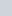), a 3-dimensional expression of the electron’s standing wave, and the binding energy (E). H represents a Hamiltonian operator, a complex quantum mechanical operator. If you’re wondering why () can’t just be cancelled from both sides, () isn’t being multiplied by a variable on the left hand side: it’s being operated on by the Hamiltonian operator. (This could be analogous to, for example, a logarithm or a square root.)

Most high school and undergraduate classes tend to not go beyond the simplified equation, but if you were to fully write out and expand the Hamiltonian operator, you would end up with the equation on the right. Within the Hamiltonian operator, h is Planck’s constant, m is mass, and V is the potential energy. The first term (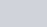) is an expression for the kinetic energy of the system. The second term (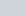) is an expression for the potential energy of the system. The third term () is an expression for the total energy of the system. Although the equation seems elaborate, it really boils down to “kinetic energy + potential energy = total energy”; i.e. conservation of Energy!

## Wave Functions and Quantum Numbers

A wave function that comes out of the solved Schrödinger equation can be written in terms of 3 quantum numbers: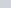,, and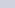. These are the quantum numbers that describe each possible spatial distribution of an electron- or in other words, an atomic orbital!, the principal quantum number, describes the average distance from the nucleus and the energy level of the orbital.
The angular momentum number (), describes the shape of the orbital. The value ofis an integer ranges from 0 to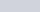. 0 corresponds to the “s” orbital, 1 corresponds to the “p” orbital, 2 corresponds to the “d” orbital, 3 corresponds to the “f”, orbital, etc.
The magnetic quantum number (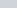), describes the orientation of the orbital. The value of m ranges from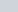to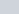.

These three quantum numbers create a kind of “coordinate system” to describe all of the orbital in an atom! For an in depth dive on quantum numbers, see this article.

## Wave Functions in Relation to Probability Density

One practical usage of the wave function was proposed by Max Born. He suggested that the probability density (probability per unit volume) of finding an electron within a certain region could be proportional to the square of the wave function (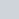). (Technically, since the wave function may contain imaginary numbers, it would be the wave function times its conjugate,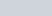, to cancel out any imaginary numbers and make the probability density positive.)

When this probability density is plotted in a graph, we can get a visual representation of an orbital. We must use probabilities because of the Heisenberg Uncertainty Principle, which states that both the speed and position of a particle cannot be precisely determined. It’s important to understand that orbitals are simply models of probability for where an electron might be, not strict confinements.

## Practice Problems

1. How many orbitals in an atom can have the quantum numbers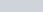and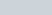?
2. What is the difference between the probability density and the probability?
3. What is the relation between probability density and the wave function?

3. The probability density is the wave function squared (().